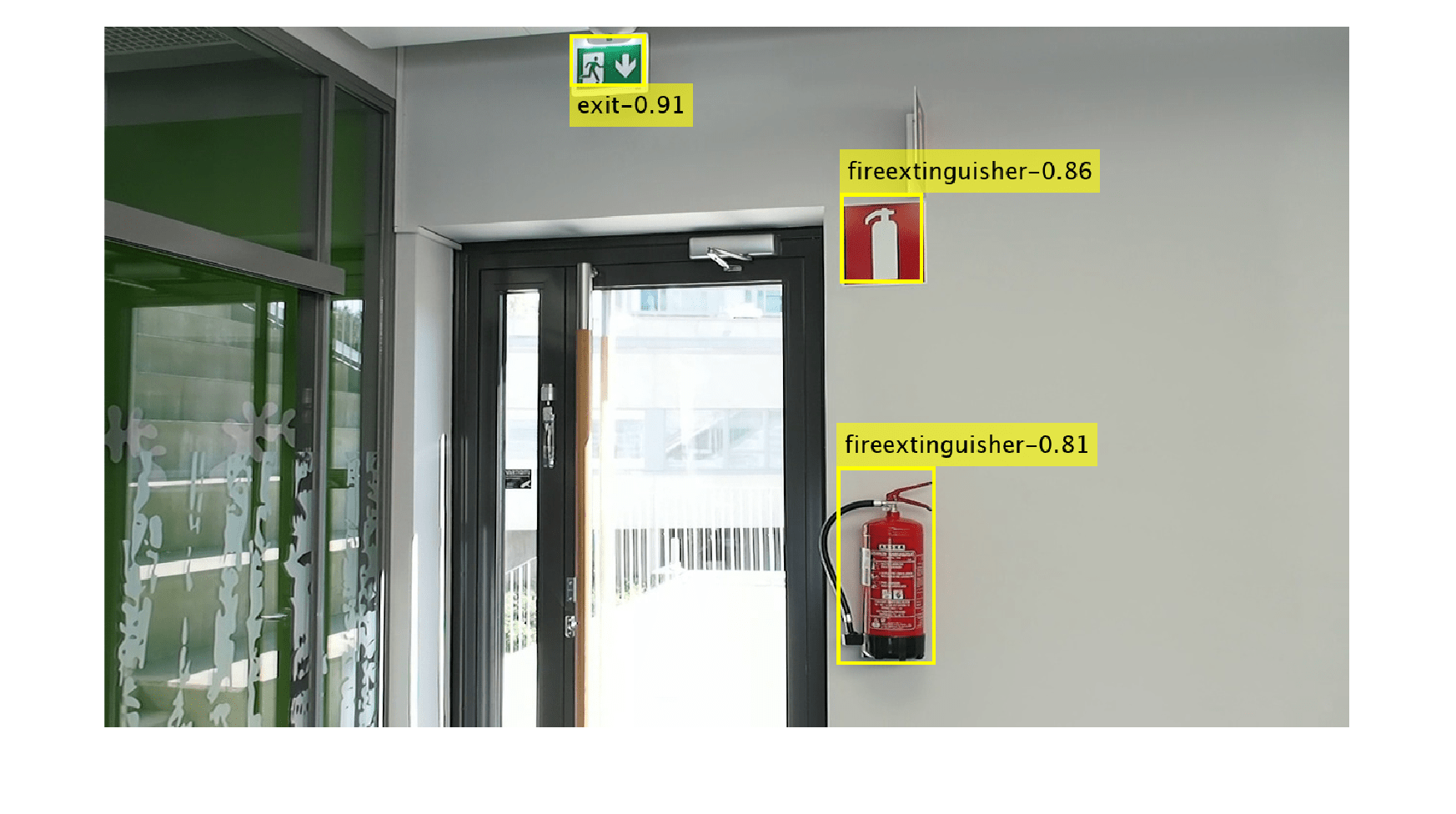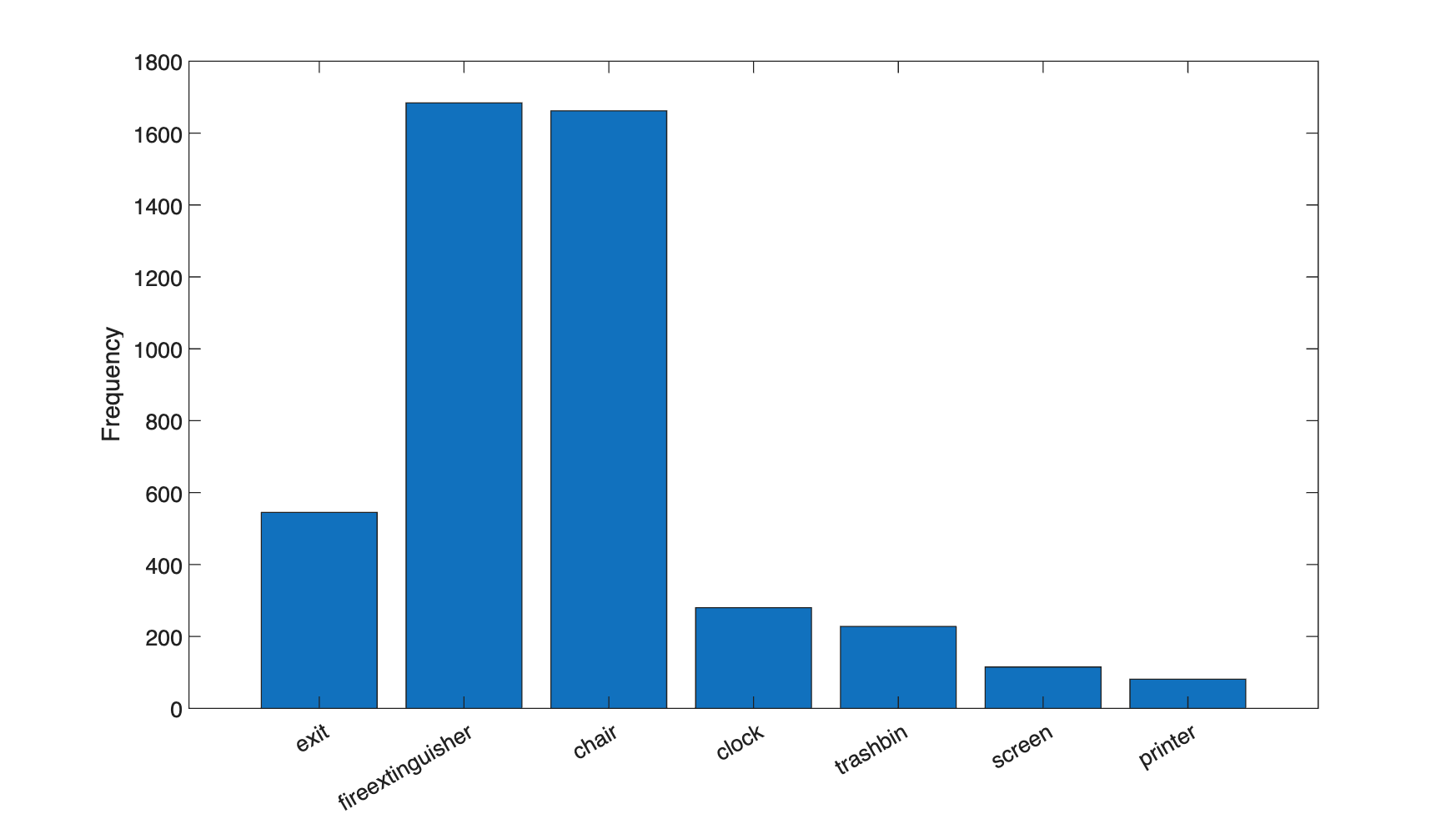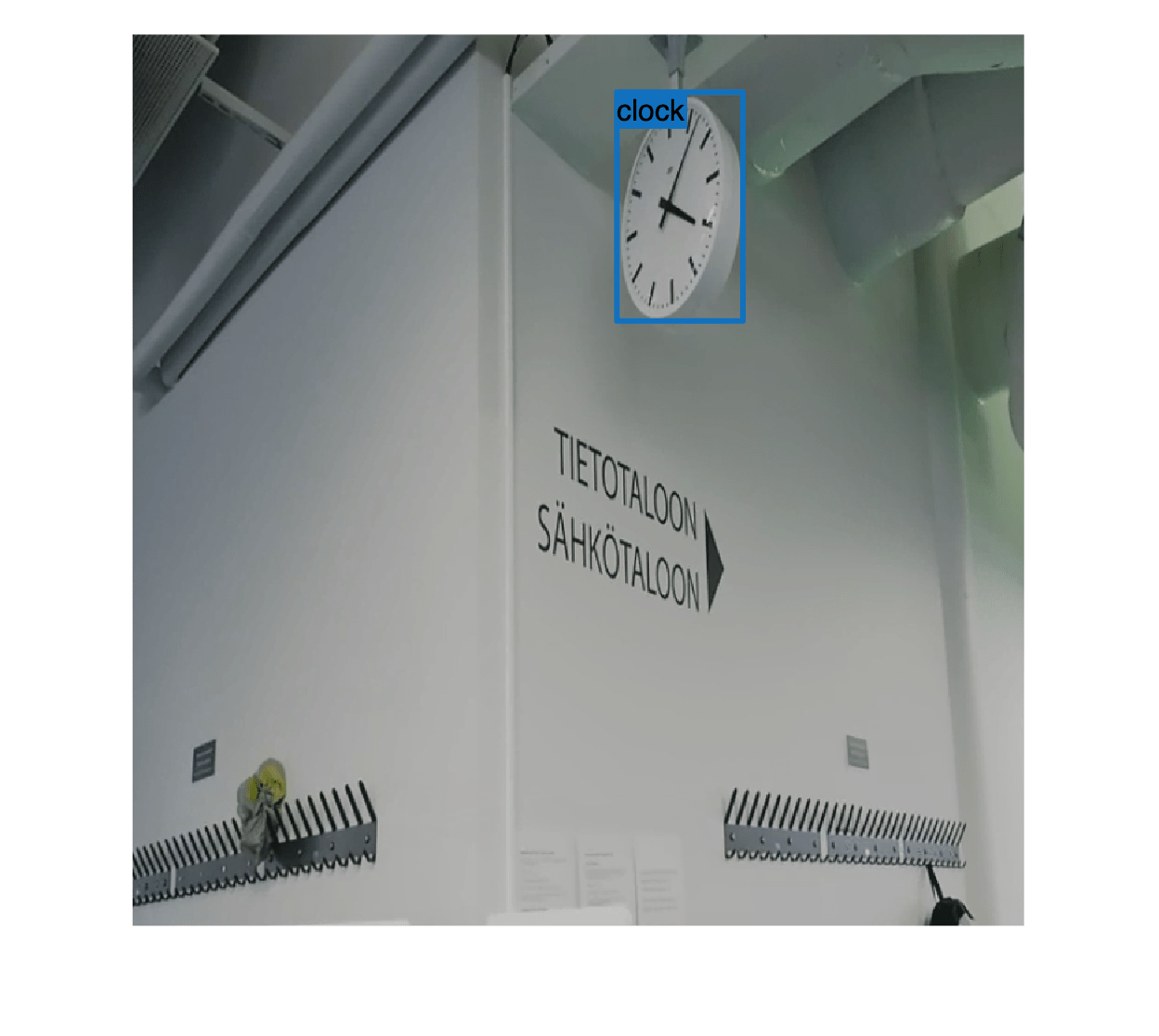# Multiclass Object Detection Using YOLO v2 Deep Learning

This example shows how to train a multiclass object detector.

### Overview

Deep learning is a powerful machine learning technique that you can use to train robust multiclass object detectors such as YOLO v2, YOLO v4, SSD, and Faster R-CNN. This example trains a YOLO v2 multiclass indoor object detector using the `trainYOLOv2ObjectDetector` function. The trained object detector is able to detect and identify multiple different indoor objects. For more information regarding training other multiclass object detectors such as YOLO v4, SSD, or Faster R-CNN, see, Getting Started with Object Detection Using Deep Learning.

### Perform Object Detection using Pretrained Detector

Read a test image that contains objects of the target classes and display it.

```I = imread('indoorTest.jpg'); imshow(I)``````pretrainedURL = "https://www.mathworks.com/supportfiles/vision/data/yolov2IndoorObjectDetector.zip"; pretrainedFolder = fullfile(tempdir,"pretrainedNetwork"); pretrainedNetworkZip = fullfile(pretrainedFolder, "yolov2IndoorObjectDetector.zip"); if ~exist(pretrainedNetworkZip,"file") mkdir(pretrainedFolder); disp("Downloading pretrained network (98 MB)..."); websave(pretrainedNetworkZip, pretrainedURL); end unzip(pretrainedNetworkZip, pretrainedFolder) pretrainedNetwork = fullfile(pretrainedFolder, "yolov2IndoorObjectDetector.mat"); pretrained = load(pretrainedNetwork); detector = pretrained.detector;```

Detect objects and their labels in the image using the `detect` function.

`[bbox, score, label] = detect(detector, I);`

Visualize the predictions by overlaying the detected bounding boxes on the image using the `insertObjectAnnotation` function.

```imshow(I) showShape("rectangle", bbox, Label=label);```This example uses the Indoor Object Detection dataset created by Bishwo Adhikari . The dataset consists of 2213 labeled images collected from indoor scenes containing 7 classes - fireextinguisher, chair, clock, trashbin, screen, and printer. Each image contains one or more labeled instances of the categories mentioned.

```dsURL = "https://zenodo.org/record/2654485/files/Indoor%20Object%20Detection%20Dataset.zip?download=1"; outputFolder = fullfile(tempdir,"indoorObjectDetection"); imagesZip = fullfile(outputFolder,"indoor.zip"); if ~exist(imagesZip,"file") mkdir(outputFolder) disp("Downloading 401 MB Indoor Objects dataset images..."); websave(imagesZip, dsURL); unzip(imagesZip, fullfile(outputFolder)); end datapath = fullfile(outputFolder, "Indoor Object Detection Dataset"); ```

The images are organized into 6 folders of different sequences. Create an `imageDatastore` by specifying the different folder paths.

```numSequences = 6; imds = imageDatastore(datapath, IncludeSubfolders=true, FileExtensions=".jpg");```

Annotations and dataset split have been provided in the file `annotationsIndoor.mat`. Load the annotations and the indices corresponding to the training, validation, and test splits. Note that the split contains 2207 images in total instead of 2213 images as 6 images have no labels associated with them. Store the indices of images containing labels in `cleanIdx`.

```data = load("annotationsIndoor.mat"); bbStore = data.BBstore; trainingIdx = data.trainingIdx; validationIdx = data.validationIdx; testIdx = data.testIdx; cleanIdx = data.idxs;```

Finally, combine the `imageDatastore` and the `boxLabelDatastore`. Split the combined datastore into train, validation and test datastores by using the `subset` command and specifying the preloaded indices.

```ds = combine(imds,bbStore); % Remove the 6 images with no labels. ds = subset(ds,cleanIdx); % Set random seed. rng(0); % Shuffle the dataset before the split to ensure good class distibution. ds = shuffle(ds); dsTrain = subset(ds,trainingIdx); dsVal = subset(ds,validationIdx); dsTest = subset(ds,testIdx); ```

### Analyze the Data

First, visualize a sample image from the dataset with the dataset.

```data = read(dsTrain); I = data{1,1}; box = data{1,2}; label = data{1,3}; imshow(I) showShape("rectangle", box, Label=label)```To measure distribution of class labels in the dataset, use `countEachLabel `to counts the number of objects by the class label.

```bbStore = ds.UnderlyingDatastores{2}; tbl = countEachLabel(bbStore)```

Visualize the counts by class.

```bar(tbl.Label,tbl.Count) ylabel("Frequency")```The classes in this dataset are unbalanced. If not handled correctly, this imbalance can be detrimental to the learning process because the learning is biased in favor of the dominant classes. There are multiple techniques used to deal will this issue - oversampling the underrepresented classes, modifying loss function, and data augmentation. You will apply data augmentation to your training data in a later section.

### Create a Yolov2 Object Detection Network

For this example, you will create a YOLO v2 object detection network. A YOLO v2 object detection network is composed of two subnetworks. A feature extraction network followed by a detection network. The feature extraction network is typically a pretrained CNN. This example uses ResNet-50 for feature extraction.

First, specify the network input size and the number of classes. When choosing the network input size, consider the minimum size required by the network itself, the size of the training images, and the computational cost incurred by processing data at the selected size. When feasible, choose a network input size that is close to the size of the training image and larger than the input size required for the network. However, reducing image resolution can make it harder for the object detector to detect smaller objects. To maintain a balance between accuracy and computational cost of running the example, specify a network input size of [450 450 3].

`inputSize = [450 450 3];`

Define number of object classes to detect.

`numClasses = 7;`

Select the base network and the feature extraction layer. Select 'activation_40_relu' as the feature extraction layer to replace the layers after 'activation_40_relu' with the detection subnetwork. This feature extraction layer outputs feature maps that are downsampled by a factor of 16. This amount of downsampling is a good trade-off between spatial resolution and the strength of the extracted features, as features extracted further down the network encode stronger image features at the cost of spatial resolution. Choosing the optimal feature extraction layer requires empirical analysis.

```network = resnet50(); featureLayer = "activation_40_relu";```

Preprocess the training data to prepare data for training. The preprocessing function will resize images and the bounding boxes. In addition, it also sanitizes the bounding boxes to convert them to a valid shape.

`preprocessedTrainingData = transform(dsTrain,@(data)resizeImageAndLabel(data, inputSize));`

Next, use estimateAnchorBoxes to estimate two anchor boxes based on the size of objects in the training data. Choosing the optimal number of anchor boxes requires empirical analysis.

```numAnchors = 2; aboxes = estimateAnchorBoxes(preprocessedTrainingData, numAnchors);```

Use the `yolov2Layers` function to create a YOLO v2 object detection network.

`lgraph = yolov2Layers(inputSize, numClasses, aboxes, network, featureLayer); `

You can visualize the network using `analyzeNetwork` or D`eepNetworkDesigner` from Deep Learning Toolbox.

### Data Augmentation

Data augmentation is used to improve network accuracy by randomly transforming the original data during training. By using data augmentation, you can add more variety to the training data without actually having to increase the number of labeled training samples. Use `transform` to augment the training data by

• Randomly flipping the image and associated box labels horizontally.

• Randomly scale the image, associated box labels.

• Jitter image color.

`augmentedTrainingData = transform(preprocessedTrainingData, @augmentData);`

Display one of the training images and box labels.

```data = read(augmentedTrainingData); I = data{1}; bbox = data{2}; label = data{3}; imshow(I) showShape("rectangle", bbox, Label=label)```### Train YOLOv2 Object Detector

Use `trainingOptions` to specify network training options.

```opts = trainingOptions("rmsprop",... InitialLearnRate=0.001,... MiniBatchSize=4,... MaxEpochs=10,... LearnRateSchedule="piecewise",... LearnRateDropPeriod=3,... VerboseFrequency=30, ... L2Regularization=0.001,... ValidationData=dsVal,... ValidationFrequency=50);```

Use `trainYOLOv2ObjectDetector` function to train YOLO v2 object detector if `doTraining` is true.

```doTraining = false; if doTraining % Train the YOLO v2 detector. [detector, info] = trainYOLOv2ObjectDetector(augmentedTrainingData,lgraph, opts); else % Load pretrained detector for the example. pretrained = load(pretrainedNetwork); detector = pretrained.detector; end```

This example was verified on an NVIDIA™ Titan X GPU with 12 GB of memory. If your GPU has less memory, you may run out of memory. If this happens, lower the `MiniBatchSize `using the `trainingOptions` function. Training this network took approximately 2 hours using this setup. Training time varies depending on the hardware you use.

### Evaluate Detector Using Test Set

Evaluate the trained object detector on test images to measure the performance. Computer Vision Toolbox™ provides object detector evaluation functions to measure common metrics such as average precision (`evaluateDetectionPrecision`) and log-average miss rates (`evaluateDetectionMissRate`). For this example, use the average precision metric to evaluate performance. The average precision provides a single number that incorporates the ability of the detector to make correct classifications (`precision`) and the ability of the detector to find all relevant objects (`recall`).

Apply the same preprocessing transform to the test data as for the training data. Note that data augmentation is not applied to the test data. Test data should be representative of the original data and be left unmodified for unbiased evaluation.

```preprocessedTestData = transform(dsTest, @(data)resizeImageAndLabel(data, inputSize)); results = detect(detector,preprocessedTestData, MiniBatchSize=4, Threshold=0.5); [ap, precision, recall] = evaluateDetectionPrecision(results, preprocessedTestData);```

The precision/recall (PR) curve highlights how precise a detector is at varying levels of recall. The ideal precision is 1 at all recall levels. The use of more data can help improve the average precision but might require more training time. Plot the PR curve for a selected class.

```classID = 1; figure plot(recall{classID},precision{classID}) xlabel("Recall") ylabel("Precision") grid on title(sprintf("Average Precision = %.2f",ap(classID)))```### Code Generation

Once the detector is trained and evaluated, you can generate code for the `yolov2ObjectDetector` using GPU Coder™. See Code Generation for Object Detection by Using YOLO v2 (GPU Coder) example for more details.

### Supporting Functions

```function B = augmentData(A) % Apply random horizontal flipping, and random X/Y scaling. Boxes that get % scaled outside the bounds are clipped if the overlap is above 0.25. Also, % jitter image color. B = cell(size(A)); I = A{1}; sz = size(I); if numel(sz)==3 && sz(3) == 3 I = jitterColorHSV(I,... Contrast=0.2,... Hue=0,... Saturation=0.1,... Brightness=0.2); end % Randomly flip and scale image. tform = randomAffine2d(XReflection=true, Scale=[1 1.1]); rout = affineOutputView(sz, tform, BoundsStyle="CenterOutput"); B{1} = imwarp(I, tform, OutputView=rout); % Sanitize boxes, if needed. This helper function is attached as a % supporting file. Open the example in MATLAB to open this function. A{2} = helperSanitizeBoxes(A{2}); % Apply same transform to boxes. [B{2},indices] = bboxwarp(A{2}, tform, rout, OverlapThreshold=0.25); B{3} = A{3}(indices); % Return original data only when all boxes are removed by warping. if isempty(indices) B = A; end end```
```function data = resizeImageAndLabel(data,targetSize) % Resize the images and scale the corresponding bounding boxes. scale = (targetSize(1:2))./size(data{1},[1 2]); data{1} = imresize(data{1},targetSize(1:2)); data{2} = bboxresize(data{2},scale); data{2} = floor(data{2}); imageSize = targetSize(1:2); boxes = data{2}; % Set boxes with negative values to have value 1. boxes(boxes<=0) = 1; % Validate if bounding box in within image boundary. boxes(:,3) = min(boxes(:,3),imageSize(2) - boxes(:,1)-1); boxes(:,4) = min(boxes(:,4),imageSize(1) - boxes(:,2)-1); data{2} = boxes; end ```

### References

 Adhikari, Bishwo; Peltomaki, Jukka; Huttunen, Heikki. (2019). Indoor Object Detection Dataset [Data set]. 7th European Workshop on Visual Information Processing 2018 (EUVIP), Tampere, Finland.